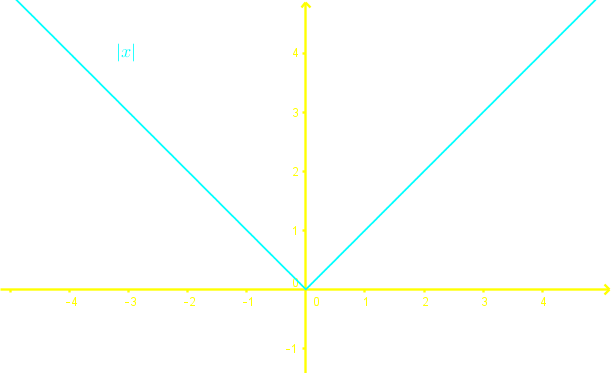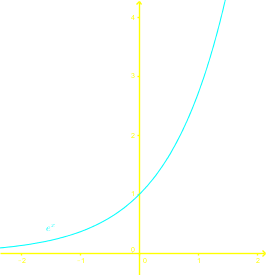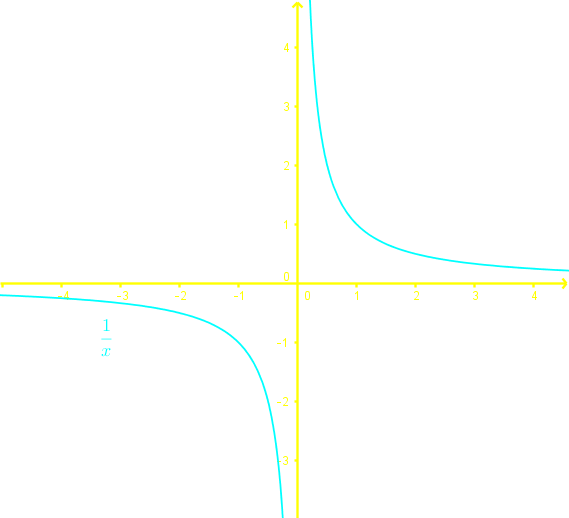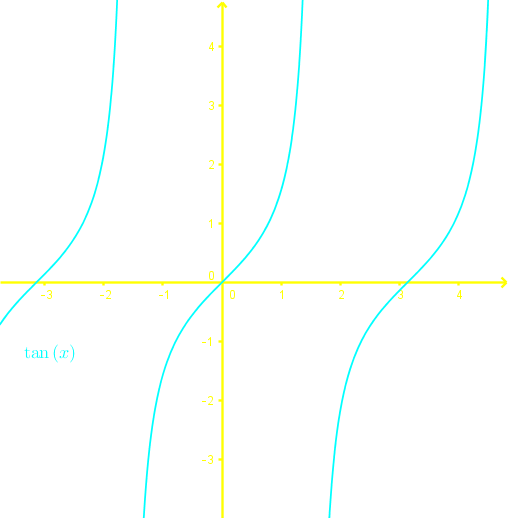# One to one functions#### Everything You Need in One Place

Homework problems? Exam preparation? Trying to grasp a concept or just brushing up the basics? Our extensive help & practice library have got you covered.#### Learn and Practice With Ease

Our proven video lessons ease you through problems quickly, and you get tonnes of friendly practice on questions that trip students up on tests and finals.#### Instant and Unlimited Help

Our personalized learning platform enables you to instantly find the exact walkthrough to your specific type of question. Activate unlimited help now!

0/1
##### Intros
###### Lessons
1. Introduction to one to one functions

i. Review: How are functions, Surjective functions and Injective functions related?

ii. How to determine if an expression is a function?

iii. What are Surjective functions?

iv. What are one to one functions?

0/3
##### Examples
###### Lessons
1. Discussing the Differences Between Surjective and Injective Functions

Identify the differences between Surjective and Injective functions and give an example for each of the functions.

1. Identifying One-to-One Functions On a Graph

Learning the Horizontal Line Test and understanding how it can be implemented to identify one-to-one functions on a graph.

1. Applying the Horizontal Line Test

Determine if the following graphs are one-to-one functions using the horizontal line test.

i.ii.iii.iv.###### Topic Notes

$\bullet$ To determine if an expression is a function, we perform the vertical line test.

$\bullet$ Surjective/Onto: For every $y$ value, there exists at least one $x$ value.

$\bullet$ Injective/Into/one-to-one: For every $y$ value, there exists at most one $x$ value.

$\bullet$ To determine if a function is one-to-one, we perform the horizontal line test.